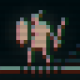```  extern number time;
vec4 effect( vec4 color, Image tex, vec2 tex_uv, vec2 pix_uv )
{
// per row offset
float f  = sin( tex_uv.y * 320.f * 3.14f );
// scale to per pixel
float o  = f * (0.35f / 320.f);
// scale for subtle effect
float s  = f * .03f + 0.97f;
float l  = sin( time * 32.f )*.03f + 0.97f;
// sample in 3 colour offset
float r = Texel( tex, vec2( tex_uv.x+o, tex_uv.y+o ) ).x;
float g = Texel( tex, vec2( tex_uv.x-o, tex_uv.y+o ) ).y;
float b = Texel( tex, vec2( tex_uv.x  , tex_uv.y-o ) ).z;
// combine as
return vec4( r*0.7f, g, b*0.9f, l ) * s;
}
```